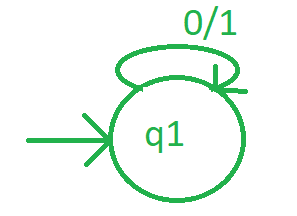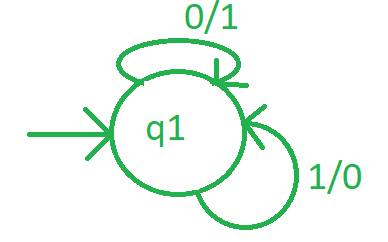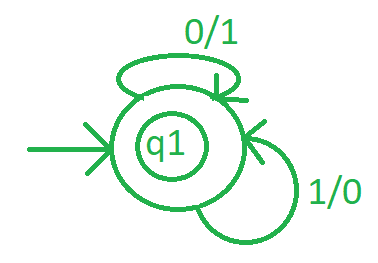Related Articles

# Mealy Machine for 1’s Complement

• Last Updated : 07 Jul, 2021

After converting a number to its binary form, replace every one of the number with 0 and every 0 with 1, then the resulting number will be known as 1, s complement of that binary number.

```Input-1 :
101010
Output-1 :
010101

Input-2 :
1110100
Output-2 :
0001011 ```

Approach :

1. First make a initial state.
2. Then convert each 0 to 1 and each 1 to 0, and reach to next possible state.
3. after performing step 2 on each digit of binary number, reach at some final state to complete the process.

Design Mealy Machine :

Step-1:
Take a initial state say q1, and if the input is found 0, convert it to 1, remain at same state and check for another input alphabet.Step-2:
If state q1 takes input alphabet is 1, then convert it to 0 and remain at same state.Step-3:As after converting all alphabet, it is on same state then this state will be final state as well.Example :
Suppose a string 10001 and start parsing from left to right. Every 0 will be replaced by 1 and every one is replaces by 0. Then the output is 01110.

Attention reader! Don’t stop learning now. Get hold of all the important CS Theory concepts for SDE interviews with the CS Theory Course at a student-friendly price and become industry ready.

My Personal Notes arrow_drop_up